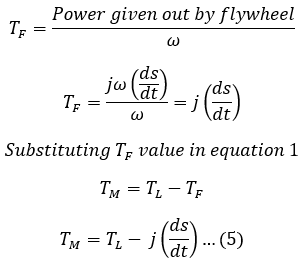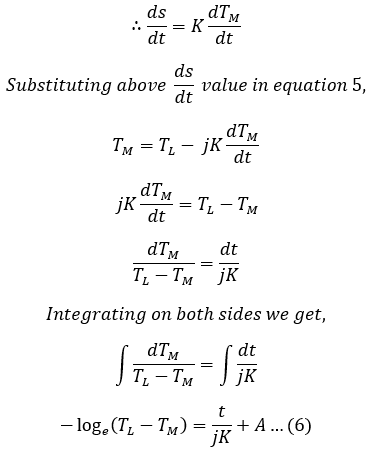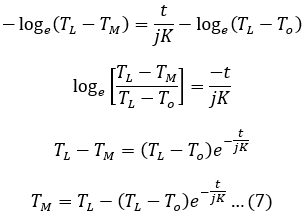# Load Equalization in Electrical Drives

The process of smoothing load fluctuations in industrial drives is called Load Equalization. In many industrial applications, the load fluctuates vary widely within short intervals of time. Electric hammers, presses, reciprocating pumps and steel billet, rolling mills are some examples of such types of loads.

When a motor is subjected to heavy loads, it will draw a large current from the supply which not only results in heavy losses but also gives rise to an appreciable voltage drop in the lines due to which the operation of other loads connected to the supply bars gets affected. Therefore it is necessary to smoothen-out load fluctuations and this process is called load equalization.

The process of load equalization for smoothing load is achieved by using a Flywheel. A flywheel is basically a heavy wheel attached to the same shaft of the motor. The flywheel acts as a reservoir that observes and redistributes stored energy.

## Function of Flywheel :

The below shows a model of the flywheel. During intervals of heavy loads, the speed of the motor decreases. Under such conditions, the flywheel releases a portion of stored kinetic energy which along with the energy drawn from the supply will meet the demand of the load.

During the light load period, the motor draws more energy from the supply than required which can be made to give out during peak periods so that the demand for the system is more or less constant.

The only requirement is that the motor should have drooping torque-speed characteristics so that there may be a drop in speed as the load comes on so as to enable the flywheel to give away its stored energy. The below curve shows the speed variations, the torque developed by the motor, and the load torque with time.

If the speed of the drive is to be reversed, such as for reversing rolling mills or as required for a colliery winder where the speed control and reversing operation is performed by the Ward-Leonard control system. In such cases, the flywheel is mounted on the shaft of the motor-generator set supplying the motor. This type of arrangement is known as the Ward-Leonard control system.

## Flywheel Calculations :

#### Consider a flywheel mounted on the shaft of the motor for load equalization.TL = Load torque (constant for load duration) in N-m,TF = Torque supplied by the flywheel in N-m,TM = Motor torque developed in N-m,To = No-load torque in N-m,ωo = No-load speed of motor in rad/sec,ω = Speed of motor in rad/sec,J = Moment of inertia of the flywheel in kg-m2,s = Slip of the motor = (ωo - ω)/ωo,t = Time in seconds.

Let the load on the motor is increasing or be at the peak load. The speed will then decrease due to which the flywheel will give out its stored kinetic energy to the load. Therefore, under this condition, the torque supplied by the motor is given as,

#### TM = TL - TF The change in kinetic energy is given by, KE = 1/2 jωo2 - 1/2 jω2KE = 1/2 j[(ωo - ω)(ωo + ω)] ...(1) Assuming that the speed drop considered is not more than 10% and because (ωo + ω)/2 is the mean speed during the interval, considering, (ωo + ω)/2 = ω ...(2)s = ωo - ω ...(3) Substituting equations 2 and 3 in equation 1 we get, KE = jωs The power given out by the flywheel will be equal to the rate of change of the energy given up by the wheel, Power given out by the flywheel = jω(ds/st) Now, the expression of torque supplied by the flywheel in terms of its moment of inertia and the drop in speed is given by,If the speed i.e., drop in speed limited to 10%, then the slip is proportional to the motor torque i.e., s ∝ TM, ∴ s = KTM Differentiating on both sides with respect to time, we get,Where A is the proportionality constant. When t = 0, the torque required will become equal to no-load torque i.e., at t = 0, TM = To. Therefore, the above equation reduces to, A = -loge(TL - To) Substituting the above value in equation 6 we get,Therefore from equation 7, it can be seen that there is an exponential rise in the motor torque and the difference between this motor torque and the load torque will give the torque produced by the flywheel.

Let the load on the motor is decreased or totally removed, due to this the motor starts accelerating and kinetic energy is stored in the flywheel. The speed of the flywheel will now start increasing up to its normal speed as the result the slip decreases making ds/dt negative. Now the motor torque will be,

## Why Flywheel Is Not Used In Synchronous Motor?

A synchronous motor is a constant speed motor that has two speeds i.e., synchronous speed and zero speed. Due to this reason, synchronous motors are employed for applications such as driving constant speed continuous mills where the load is for relatively long periods.

When we go on increasing the excitation of the motor or the generated voltage, the load torque or power developed to pull it out also becomes large.

Due to this reason, large overloads can be applied to a synchronous motor for a long period of time and the motor itself performs the compensation action for the variation of driving loads and consequently carries the entire load. Therefore flywheel is not used with a synchronous motor for load equalization.

Do not enter any spam links and messages